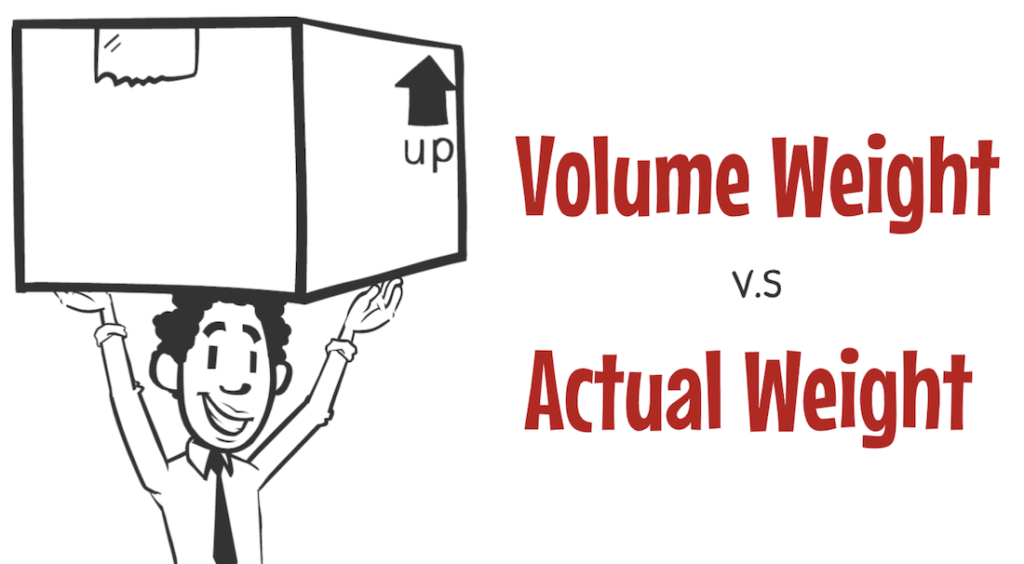Senior Cat

About difference between Actual Weight and Volume WeightSenior Seagull

This is 5:00 viedeo.

Today, I would like to explain the difference between volume weight and actual weight in logistics.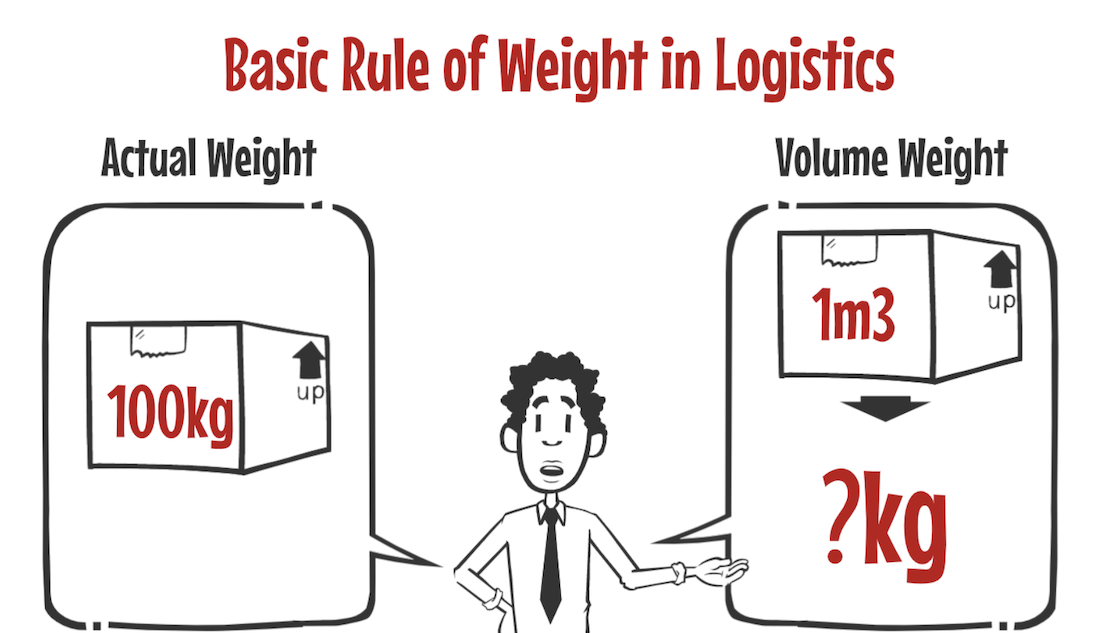First, what are actual weight and volume weight?
These are the technical terms for basic weight in the logistics industry.

Actual weight is literally actual weight, and volume weight is the weight of a cargo, when the size of the cargo is converted into weight.

Actual weight is understandable, but volume weight is difficult to understand, isn’t it?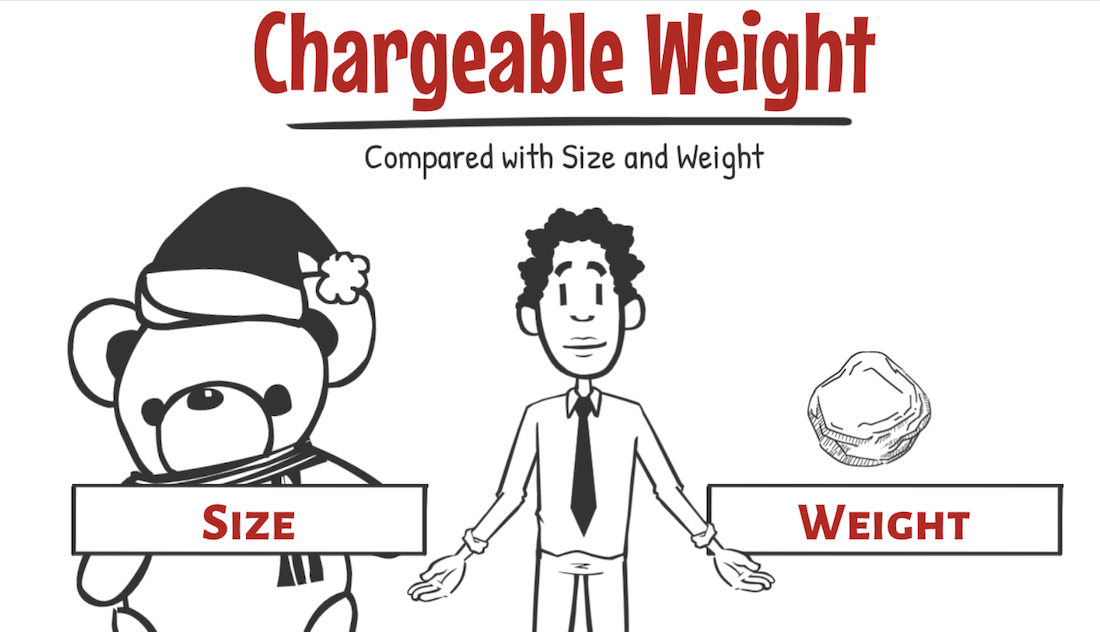To understand volume weight, let’s learn about Chargeable Weight which is commonly used in logistics jobs. So I would like to explain it together.

Chargeable Weight is described by comparing the size and weight of a cargo, and using the greater one for cost calculation. The point is to compare the different units, “large and heavy” to each other.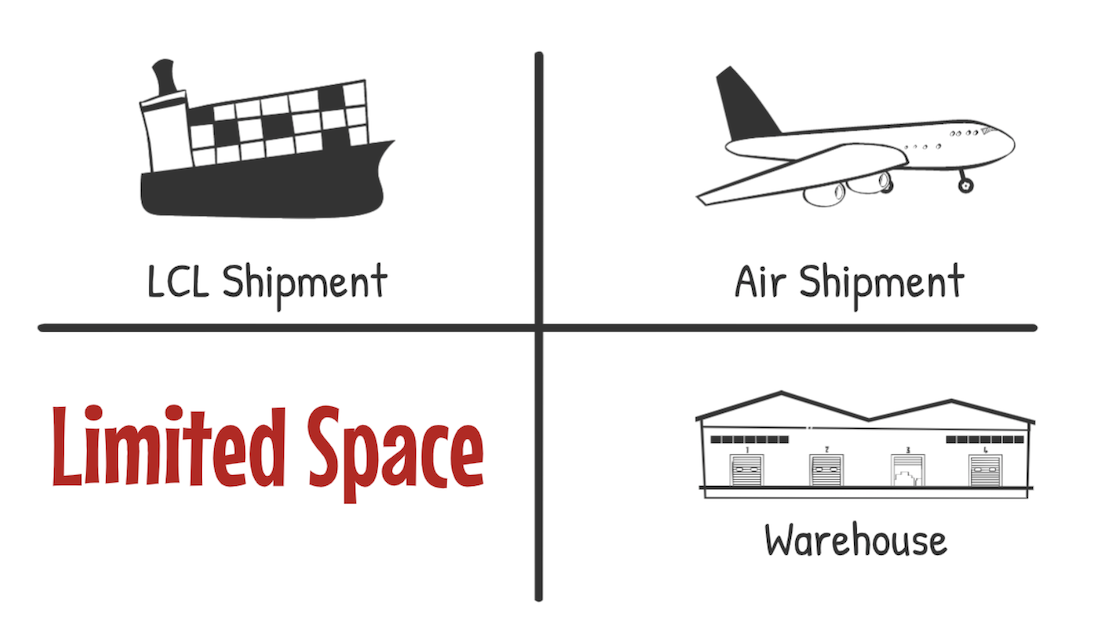Logistics uses a limited amount of space to deliver and store cargos. The reason is like this. when you do business with limited space, such as shipping lines, airlines, and warehouse companies.

It may not be fair if you use only one unit of size or weight for cost calculation.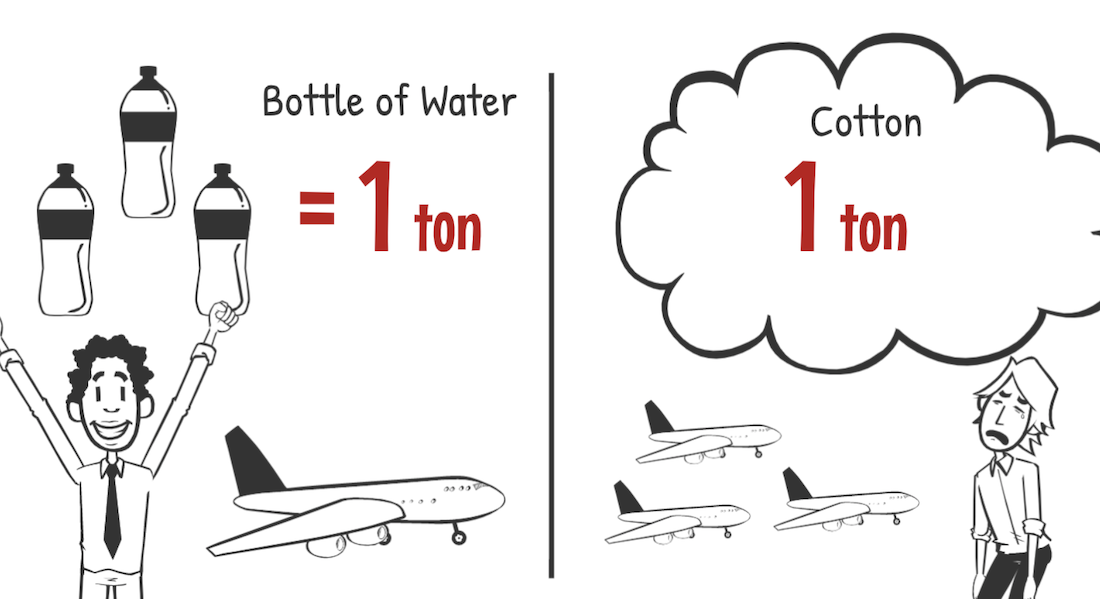Let me give you an example. For example, if you are transporting 1 ton of bottle of water and 1 ton of cotton by air shipment, which one will take up more space?

Water is heavy but not bulky. But cotton is light and very bulky, so it takes up a lot of space.

Air line will have a big loss if they do cost calculation by actual weight for 1 ton of cotton, because transporting the bulky cotton takes up a lot of space in the airplane.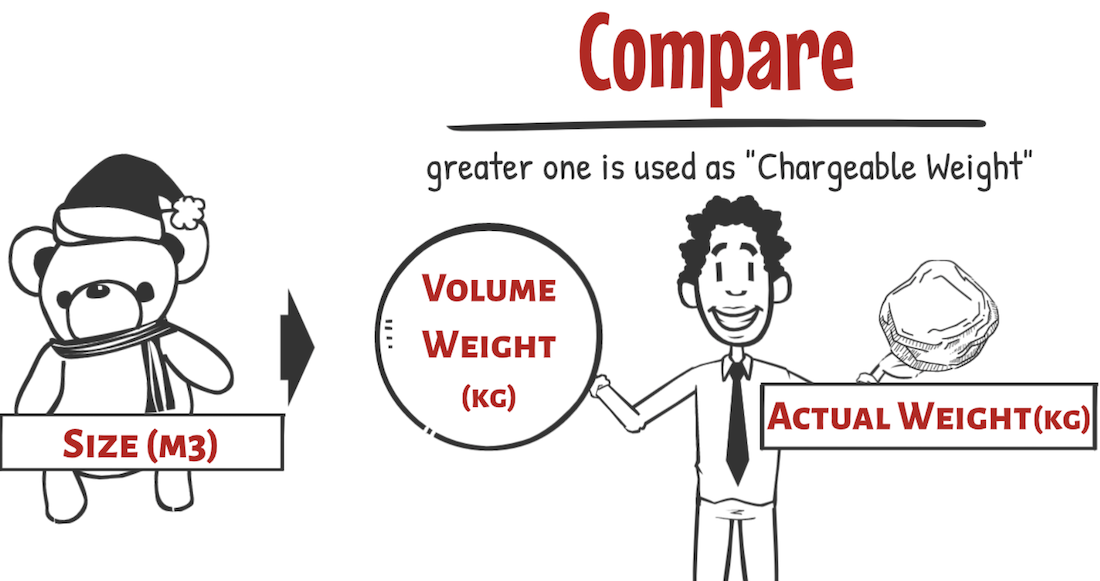To prevent this, volume weight is used for cost calculation as well. Actual weight and volume weight are compared, and the greater one is used as Chargeable Weight.

Let’s actually compare actual weight and volume weight.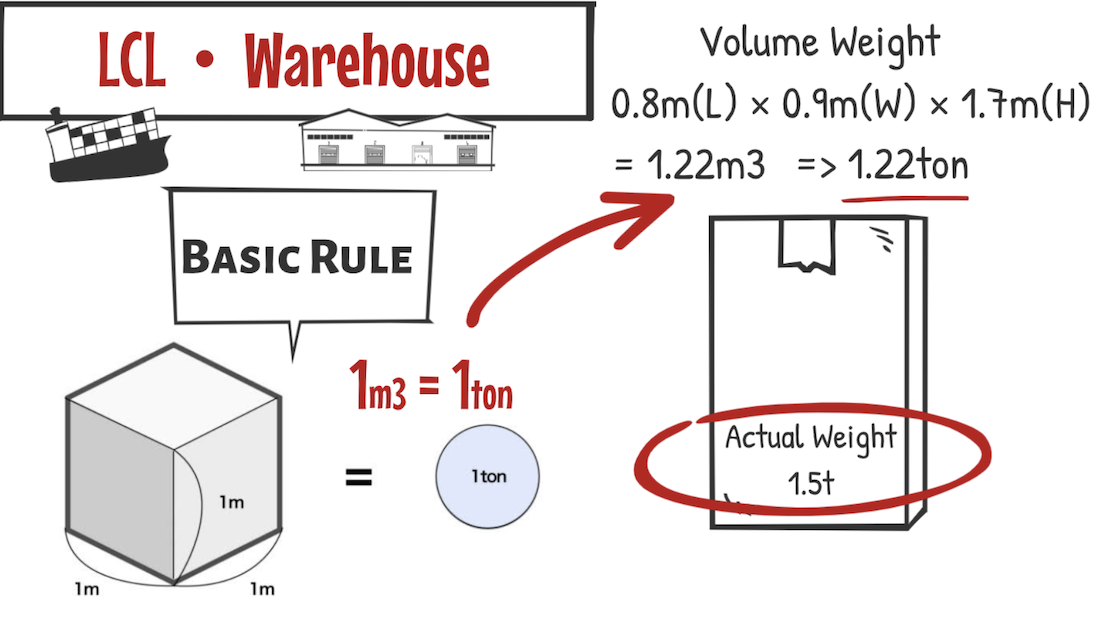First of all, for LCL shipment and warehouse. There is a rule to calculate volume weight.
The rule is “1 cubic meter = 1 ton.” You absolutely remember this.

For example, if you have a cargo with an actual weight of 1.5 tons, suppose this cargo size is 0.8meter long, 0.9meter wide and 1.7meter high. The volume of this cargo is 1.22 cubic meters.

If we use the earlier rule that one cubic meter is one ton, 1.22 cubic meters is equal to 1.22 tons.
And the actual weight is 1.5 tons and the volume weight is 1.22 tons.

In this case, the larger one (chargeable weight) is 1.5 tons, the actual weight.Next, let’s look at how to calculate volume weight in air transportation.
In air transportation, it’s different from the rules for marine transport and warehousing.

Volume (cm3) is divided by 6,000 = volume weight (kg).
One thing to note is the units.

Unlike the previous example, cubic centimeters and kilograms are commonly used for air transportation.
Some courier companies may divide by 5,000 instead of 6,000, but 6,000 is more common.

Let me give you an example.

Suppose a cargo has actual weight of 50 kg, and cargo size of 70 cm long, 90 cm wide, and 90 cm high.
The volume of this cargo is 567,000 cubic centimeters, so it becomes 94.5 kg when divided at 6,000.

Actual weight is 50kg and volume weight is 94.5kg, which means Chargeable Weight is 94.5kg, volume weight.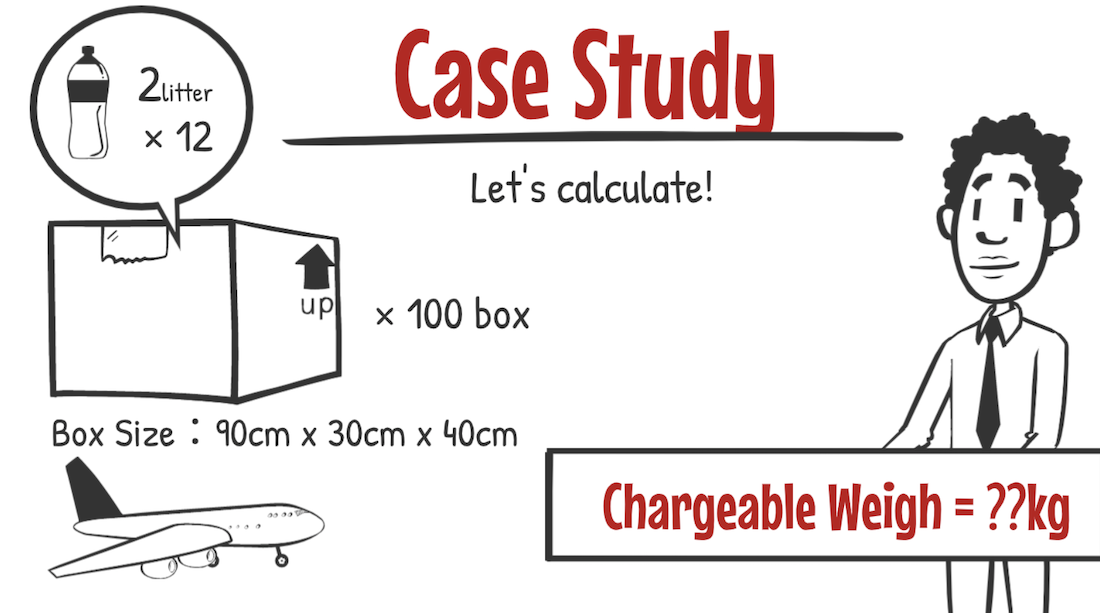Finally, let’s do a case study. There is a box containing 12 bottles of 2 liters of water. We plan to ship 100 boxes of these by air shipment. The size of the box is 90 cm long, 30 cm wide and 40 cm high.

What would the Chargeable Weight of this shipment be?
The answer is in the summary column of the video, so try to calculate it once.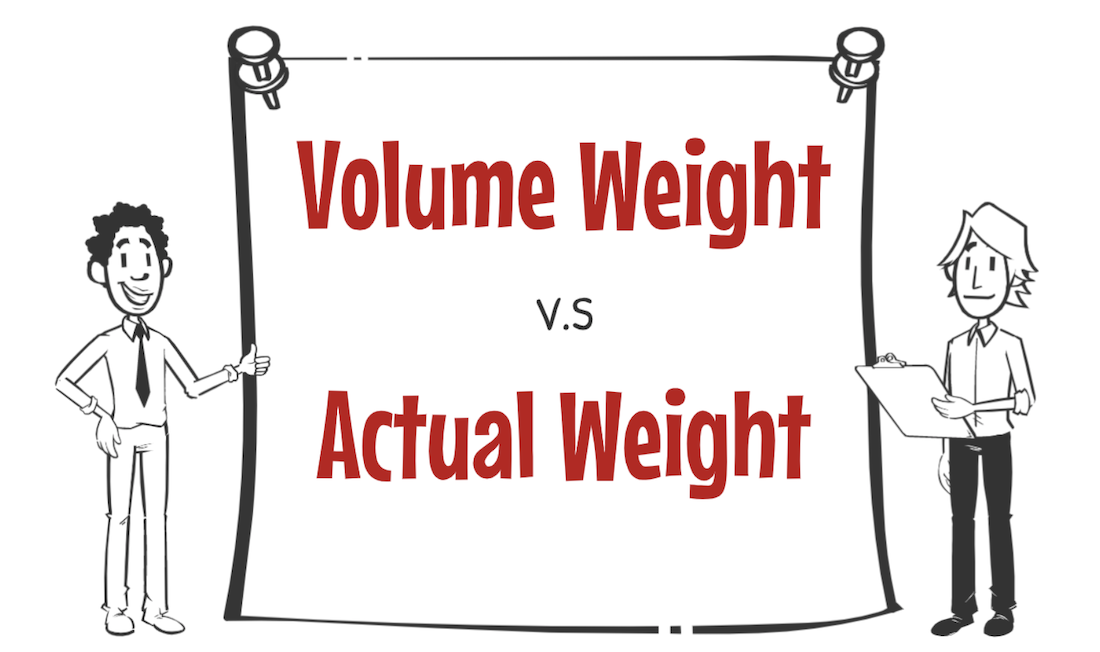How did you like it? Today, I explained the difference between volume weight and actual weight.This is basic word in the logistics industry, And you have to remember the calculation method as a rule.

It is frequently used in LCL, air transport, warehouse and trucking practices.
Please understand the contents of this video and you’ll be able to use it.

## Contact to IINO san★Contact to IINO san★
—————————————–IINO